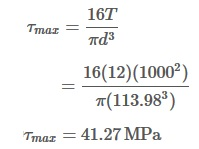Problem 302:
What is the minimum diameter of a solid steel shaft that will not twist through more than 3° in a 6-m length when subjected to a torque of 12 kN·m? What maximum shearing stress is developed? Use G = 83 GPa.

Solution:

Given,
Length, L = 6 m
Torque, T = 12 KN.m
Angle of twist, θ = 3°
G = 83 GPa.
Minimum diameter, d =?
Maximum shearing stress, τmax =?

We know that , Angle of twist,So, Minimum diameter = 113.98 mm

We also know that, maximum shearing stress,So, Maximum shearing stress = 41.27 MPa